# Probability of large deviations

(diff) ← Older revision | Latest revision (diff) | Newer revision → (diff)

A probability of the type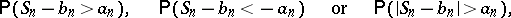where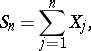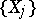is a sequence of independent random variables, and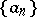and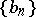are two numerical sequences such that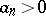, andin probability.

If the random variables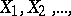have the same distribution with mathematical expectation zero and finite variance, one may write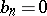and, whereas. Cramér's theorem and strengthened versions of it are particularly important in this connection (cf. Cramér theorem).

To obtain guaranteed bounds for the probability of large deviations one uses inequalities of the type of the Chebyshev inequality in probability theory; these provide the so-called exponential bounds for the probability of large deviations. For instance, if the random variables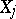are independent,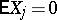,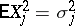,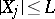with probability one,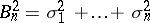and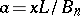, then the estimate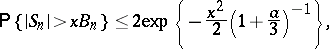the right-hand side of which decreases exponentially with increasing, is valid for all.

How to Cite This Entry:
Probability of large deviations. Encyclopedia of Mathematics. URL: http://encyclopediaofmath.org/index.php?title=Probability_of_large_deviations&oldid=16113
This article was adapted from an original article by V.V. PetrovV.V. Yurinskii (originator), which appeared in Encyclopedia of Mathematics - ISBN 1402006098. See original article